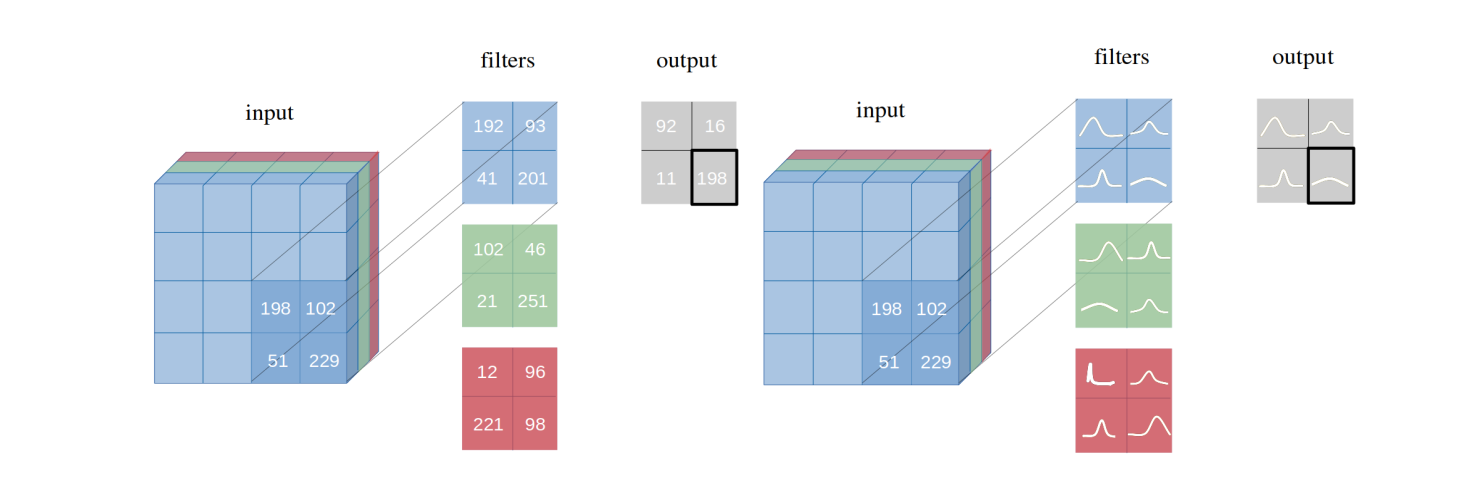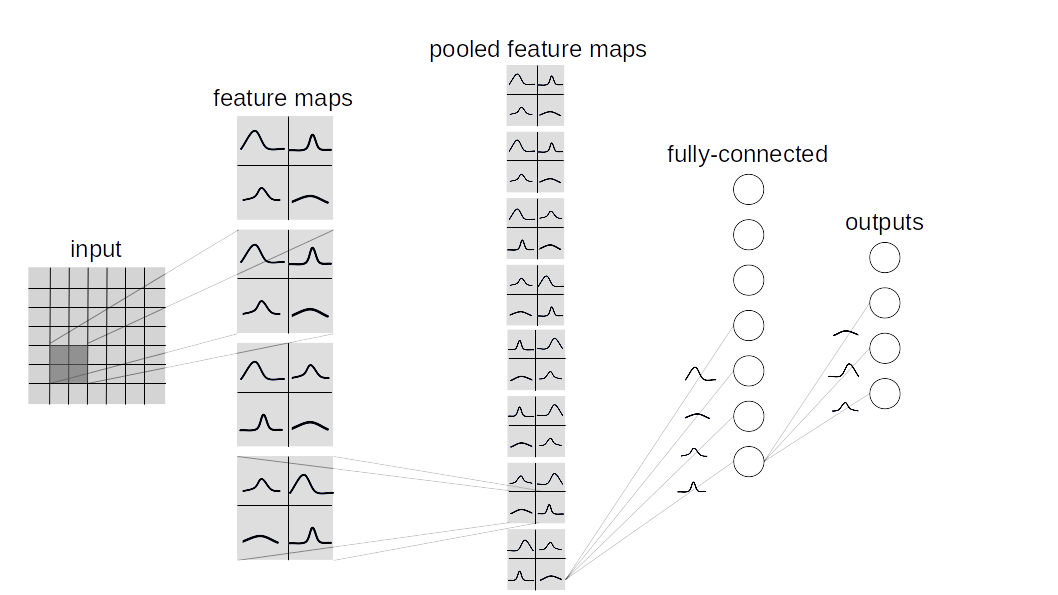We introduce Bayesian convolutional neural networks with variational inference, a variant of convolutional neural networks (CNNs), in which the intractable posterior probability distributions over weights are inferred by Bayes by Backprop. We demonstrate how our proposed variational inference method achieves performances equivalent to frequentist inference in identical architectures on several datasets (MNIST, CIFAR10, CIFAR100) as described in the paper.

### Filter weight distributions in a Bayesian Vs Frequentist approach### Fully Bayesian perspective of an entire CNN### Layer types

This repository contains two types of bayesian lauer implementation:

• BBB (Bayes by Backprop):
Based on this paper. This layer samples all the weights individually and then combines them with the inputs to compute a sample from the activations.

• BBB_LRT (Bayes by Backprop w/ Local Reparametrization Trick):
This layer combines Bayes by Backprop with local reparametrization trick from this paper. This trick makes it possible to directly sample from the distribution over activations.

### Make your custom Bayesian Network?

To make a custom Bayesian Network, inherit `layers.misc.ModuleWrapper` instead of `torch.nn.Module` and use `BBBLinear` and `BBBConv2d` from any of the given layers (`BBB` or `BBB_LRT`) instead of `torch.nn.Linear` and `torch.nn.Conv2d`. Moreover, no need to define `forward` method. It'll automatically be taken care of by `ModuleWrapper`.

For example:

``````class Net(nn.Module):

def __init__(self):
super().__init__()
self.conv = nn.Conv2d(3, 16, 5, strides=2)
self.bn = nn.BatchNorm2d(16)
self.relu = nn.ReLU()
self.fc = nn.Linear(800, 10)

def forward(self, x):
x = self.conv(x)
x = self.bn(x)
x = self.relu(x)
x = x.view(-1, 800)
x = self.fc(x)
return x
``````

Above Network can be converted to Bayesian as follows:

``````class Net(ModuleWrapper):

def __init__(self):
super().__init__()
self.conv = BBBConv2d(3, 16, 5, strides=2)
self.bn = nn.BatchNorm2d(16)
self.relu = nn.ReLU()
self.flatten = FlattenLayer(800)
self.fc = BBBLinear(800, 10)
``````

#### Notes:

1. Add `FlattenLayer` before first `BBBLinear` block.
2. `forward` method of the model will return a tuple as `(logits, kl)`.
3. `priors` can be passed as an argument to the layers. Default value is:
``````priors={
'prior_mu': 0,
'prior_sigma': 0.1,
'posterior_mu_initial': (0, 0.1),  # (mean, std) normal_
'posterior_rho_initial': (-3, 0.1),  # (mean, std) normal_
}
``````

### How to perform standard experiments?

Currently, following datasets and models are supported.

• Datasets: MNIST, CIFAR10, CIFAR100
• Models: AlexNet, LeNet, 3Conv3FC

#### Bayesian

`python main_bayesian.py`

• set hyperparameters in `config_bayesian.py`

#### Frequentist

`python main_frequentist.py`

• set hyperparameters in `config_frequentist.py`

### Directory Structure:

`layers/`: Contains `ModuleWrapper`, `FlattenLayer`, `BBBLinear` and `BBBConv2d`.
`models/BayesianModels/`: Contains standard Bayesian models (BBBLeNet, BBBAlexNet, BBB3Conv3FC).
`models/NonBayesianModels/`: Contains standard Non-Bayesian models (LeNet, AlexNet).
`checkpoints/`: Checkpoint directory: Models will be saved here.
`tests/`: Basic unittest cases for layers and models.
`main_bayesian.py`: Train and Evaluate Bayesian models.
`config_bayesian.py`: Hyperparameters for `main_bayesian` file.
`main_frequentist.py`: Train and Evaluate non-Bayesian (Frequentist) models.
`config_frequentist.py`: Hyperparameters for `main_frequentist` file.

### Uncertainty Estimation:

There are two types of uncertainties: Aleatoric and Epistemic.
Aleatoric uncertainty is a measure for the variation of data and Epistemic uncertainty is caused by the model.
Here, two methods are provided in `uncertainty_estimation.py`, those are `'softmax'` & `'normalized'` and are respectively based on equation 4 from this paper and equation 15 from this paper.
Also, `uncertainty_estimation.py` can be used to compare uncertainties by a Bayesian Neural Network on `MNIST` and `notMNIST` dataset. You can provide arguments like:

1. `net_type`: `lenet`, `alexnet` or `3conv3fc`. Default is `lenet`.
2. `weights_path`: Weights for the given `net_type`. Default is `'checkpoints/MNIST/bayesian/model_lenet.pt'`.
3. `not_mnist_dir`: Directory of `notMNIST` dataset. Default is `'data\'`.
4. `num_batches`: Number of batches for which uncertainties need to be calculated.

Notes:

2. Parameters `layer_type` and `activation_type` used in `uncertainty_etimation.py` needs to be set from `config_bayesian.py` in order to match with provided weights.

If you are using this work, please cite:

``````@article{shridhar2019comprehensive,
title={A comprehensive guide to bayesian convolutional neural network with variational inference},
author={Shridhar, Kumar and Laumann, Felix and Liwicki, Marcus},
journal={arXiv preprint arXiv:1901.02731},
year={2019}
}
``````
``````@article{shridhar2018uncertainty,
title={Uncertainty estimations by softplus normalization in bayesian convolutional neural networks with variational inference},
author={Shridhar, Kumar and Laumann, Felix and Liwicki, Marcus},
journal={arXiv preprint arXiv:1806.05978},
year={2018}
}
}
``````

Get A Weekly Email With Trending Projects For These Topics
No Spam. Unsubscribe easily at any time.
Python (888,878
Pytorch (22,609
Convolutional Neural Networks (12,853
Bayesian (3,725
Image Recognition (1,157
Bayesian Inference (873
Variational Inference (363
Bayesian Statistics (320
Bayesian Network (137
Pytorch Cnn (104
Bayes (93
Bayesian Neural Networks (67
Bayesian Deep Learning (55
Variational Bayes (35
Bayes By Backprop (4
Aleatoric Uncertainties (3
Bayesian Convnets (3